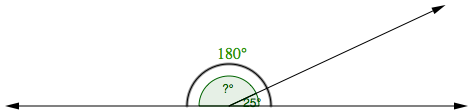### Home > MC1 > Chapter 9 > Lesson 9.3.2 > Problem9-143

9-143.

Two angles combine to form a straight line. One of the angles measures $25°$. What is the measure of the other angle? Show or explain how you found your answer.

Look at the diagram below. What is the measure of the angles when they are combined? What is the measure of the other angle?